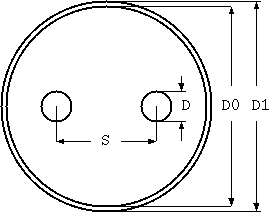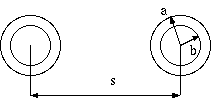# Proximity effect of parallel circular conductors computation

## 1. parallel circular solid conductorsconductor diameter (D mm)
conductor spacing (S mm)
shield innter diameter (D0 mm)
shield outer diameter (D1 mm)
frequency (Hz)
conductor conductivity (1.0 for annealed copper conductor)
conductor permeability (1.0 for vacuum)
shield conductivity (1.0 for annealed copper conductor
shield permeability (1.0 for vacuum)
If conductor shield inner diameter is set larger enough or set to zero, it will be in the case of unshielded. If conductivity and permeability is set to zero, shield is assumed to be the same material as the conductor material.

## 2. Parallel solid or tubular conductorsconductor outer diameter (a mm)
conductor inner diameter (b mm)
conductor spacing (s mm)
frequency (Hz)
If inner diameter is set to zero, this will be the solid case.

## Commentary

If we want to calculate the AC resistance and inductance of multi-core cable,

1. skin effect
2. proximity effect
3. eddy current in other surrounding conductors
must be considerd. Here are simple but impotant cases frequently used in many design.

Surrounding conductors increase AC resistance and decrease inductance.

The first calculation is based on

```  V.Belevitch,- Theory of Proximity Effect in Multiwair Cables
(Philips Res. Repts, 32, 16-43/96-117, 1977)
```

The second calculation is based on

```  A.H.M.Arnold,- Proximity Effect in Solid and Hollow Round Conductors
(Jour. I.E.E. vol 88, Part 2, 349-359, 1941)
```

In addition, the permeability of the air is 4e-7 * π (H / m), and condctivity of copper is 5.8e7 (G / m)

1999-04-20, Kouichi Hirabayashi